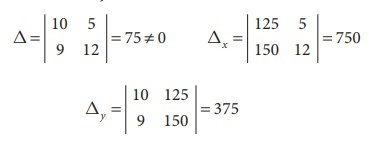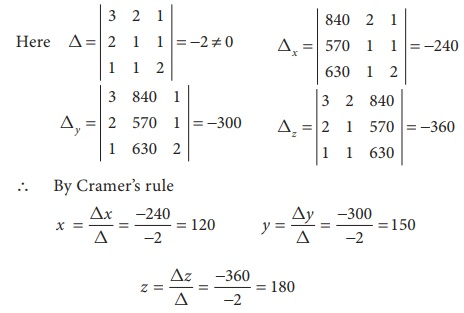Home | | Business Maths 12th Std | CramerŌĆÖs Rule - Non Homogeneous linear equations upto three variables: Solved Example Problems

# CramerŌĆÖs Rule - Non Homogeneous linear equations upto three variables: Solved Example Problems

Business Maths and Statistics : Applications of Matrices and Determinants: CramerŌĆÖs Rule - Non Homogeneous linear equations upto three variables: Solved Example Problems with Answers, Solution and Explanation

## CramerŌĆÖs Rule (Non Homogeneous linear equations upto three variables): Solved Example Problems

Example 1.19

Solve the equations 2x + 3y = 7, 3x + 5y = 9 by CramerŌĆÖs rule.

Solution:

The equations are

2x + 3y = 7

3x + 5y = 9

HereŌł┤ we can apply CramerŌĆÖs RuleŌł┤ By CramerŌĆÖs ruleŌł┤    Solution is      = 8, y = ŌłÆ3

Example 1.20

The following table represents the number of shares of two companies A and B during the month of January and February and it also gives the amount in rupees invested by Ravi during these two months for the purchase of shares of two companies. Find the the price per share of A and Bpurchased during both the monthsSolution:

Let the price of one share of A be x

Let the price of one share of B be y

Ōł┤ By given data, we get the following equations

10x + 5y = 125

9x + 12 y = 150Ōł┤ By CramerŌĆÖs ruleThe price of the share A is Ōé╣10 and the price of the share B is Ōé╣5.

Example 1.21

The total cost of 11 pencils and 3 erasers is Ōé╣ 64 and the total cost of 8 pencils and 3 erasers is Ōé╣49. Find the cost of each pencil and each eraser by CramerŌĆÖs rule.

Solution:

Let ŌĆśxŌĆÖ be the cost of a pencil

Let ŌĆśyŌĆÖ be the cost of an eraser

Ōł┤ By given data, we get the following equations

11x + 3y =64

8x+3y=49Ōł┤ The cost of a pencil is Ōé╣ 5 and the cost of an eraser is Ōé╣ 3.

Example 1.22

Solve by CramerŌĆÖs rule x + y + z = 4, 2x ŌłÆ y + 3z = 1, 3x + 2 y ŌłÆ z = 1

Solution:Ōł┤ We can apply CramerŌĆÖs Rule and the system is consistent and it has unique solution.The solution is     ( x , y, z) = ( ŌłÆ1,3, 2)

Example 1.23

The price of 3 Business Mathematics books, 2 Accountancy books and one Commerce book is Ōé╣840. The price of 2 Business Mathematics books, one Accountancy book and one Commerce book is Ōé╣570. The price of one Business Mathematics book, one Accountancy book and 2 Commerce books is Ōé╣630. Find the cost of each book by using CramerŌĆÖs rule.

Solution:

Let ŌĆśxŌĆÖ be the cost of a Business Mathematics book

Let ŌĆśyŌĆÖ be the cost of a Accountancy book.

Let ŌĆśzŌĆÖ be the cost of a Commerce book.

Ōł┤ 3x + 2 y + z = 840

2x + y + z = 570

+ y + 2= 630Ōł┤ The cost of a Business Mathematics book is Ōé╣120,

the cost of a Accountancy book is Ōé╣150 and

the cost of a Commerce book is Ōé╣180.

Example 1.24

An automobile company uses three types of Steel S1S 2 and S3 for providing three different types of Cars C1C2 and C3. Steel requirement R (in tonnes) for each type of car and total available steel of all the three types are summarized in the following table.Determine the number of Cars of each type which can be produced by CramerŌĆÖs rule.

Solution:

Let ŌĆśxŌĆÖ be the number of cars of type C1

Let ŌĆśyŌĆÖ be the number of cars of type C2

Let ŌĆśzŌĆÖ be the number of cars of type C3

3x + 2 y + 4z = 28

+ y + 2= 13

2x + 2 y + z = 14Ōł┤ The number of cars of each type which can be produced are 2, 3 and 4.

Study Material, Lecturing Notes, Assignment, Reference, Wiki description explanation, brief detail
12th Business Maths and Statistics : Chapter 1 : Applications of Matrices and Determinants : CramerŌĆÖs Rule - Non Homogeneous linear equations upto three variables: Solved Example Problems |